Teach
With
Bigshot
 Prev Introduction      Pinhole      Refraction      Lens Basics      Thin Lens Lens Properties      Focusing      Image Appearance      Illustrations Next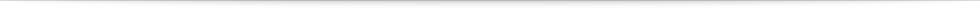### How Does a Lens Work?

Lenses are usually made of glass or plastic, and use refraction to either converge (funnel) or diverge (spread) the light that they receive. Depending on the application, lens makers carefully design the shape of the lens to achieve the right amount of convergence or divergence.

Most lenses are spherical − that is, each of their surfaces is a part of a sphere. Lenses that bulge outwards are called convex lenses and ones that cave in are called concave lenses. As shown in Figure 7, convex lenses converge light and concave lenses diverge light.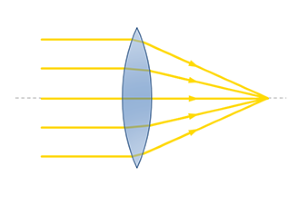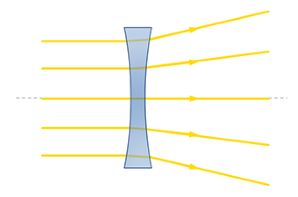Figure 7a: A convex lens converges light Figure 7b: A concave lens diverges light

Sometimes a single lens may not be enough to achieve the kind of bending we need. In such cases, several lenses of different shapes and sizes may be used to form a compound lens.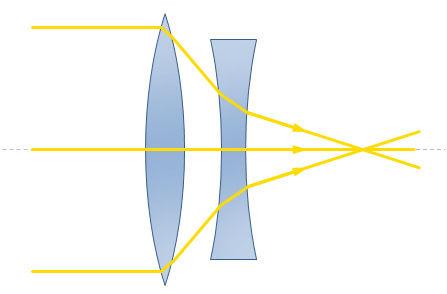Figure 8: A compound lens comprised of a convex and a concave lens

While modern cameras use compound lenses with several individual lenses with complex shapes to capture high quality images, their working principle can still be described using a single thin convex lens. Let’s take a closer look at how a thin lens works.

 Prev Next###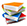References

  "Lens_(Optics)," Wikipedia, The Free Encyclopedia. Oct 2, 2009. [Online]. Available: http://en.wikipedia.org/wiki/Lens_(optics). [Accessed: Oct 4, 2009].